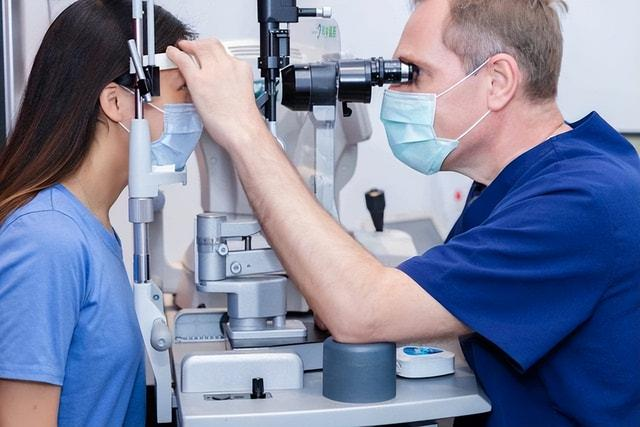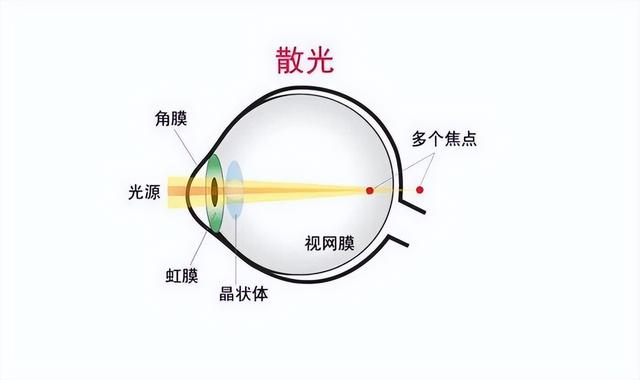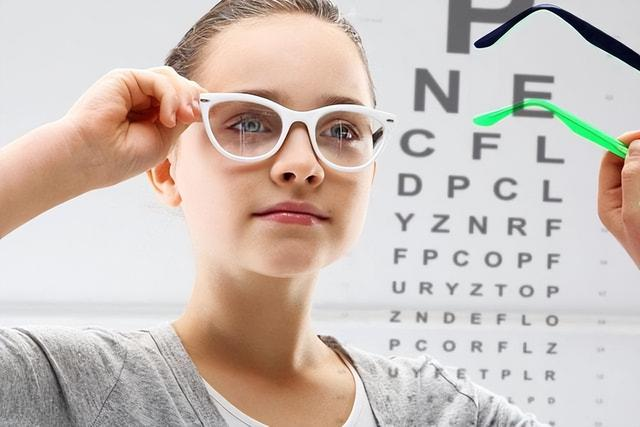# Teach you how to read the refraction report

Refractive examination report includes four parts: spherical power, cylinder power, axial position of cylinder power and corrected vision. The spherical power can be used to know whether it is hyperopia or myopia, and the cylindrical power can be used to know whether it is farsighted or myopic astigmatism. The axis of the cylindrical power represents the direction of astigmatism.

With the popularity of various electronic products, more and more people are suffering from nearsightedness, farsightedness or astigmatism, all of which are refractive errors. Refractive errors can cause vision loss and have a certain impact on normal life. You should go to the hospital for a refractive examination to understand the detailed condition. So what about the refraction report?1, Cylinder power

Cylinder power is refraction inspection The second item of the report is generally marked with a capital letter “C”. For example, there is a (+) in front of the cylinder degree, which represents the degree of hyperopia astigmatism; there is a (-) in front of the cylinder degree, which represents the degree of myopia astigmatism. The degree of detail should be the number multiplied by 100. If the number is -1.0, it represents 100 degrees of myopia astigmatism, and if the number is +1.0, it represents 100 degrees of hyperopia astigmatism.2, Cylinder Power Axial

Most refraction tests The content on the far right of the report is the axial position of the cylinder power, that is, the axial position of astigmatism, which is marked with a capital letter “A”. The axial position represents the direction of astigmatism, which is a semicircle degree, from 0 degrees to 180 degrees, so the difference between the axial positions is small, and the axial position of 5 and 180 are almost the same.

3. Spherical degree

Spherical degree Generally, it is marked with a capital letter “S”. If there is a (+) before the spherical lens degree, it represents hyperopia; there is a (-) before the spherical lens degree, it represents myopia. The degree of detail is the number multiplied by 100. If the number is -1.50, it represents 150 degrees of myopia, and the number is +2.75, which represents 275 degrees of hyperopia.

4. Corrected vision

If it is comprehensive optometry The report usually includes corrected vision. Corrected visual acuity is the visual acuity after wearing glasses. For example, if the report shows that the corrected visual acuity is 1.0, it means that the patient’s visual acuity after wearing glasses is 1.0.